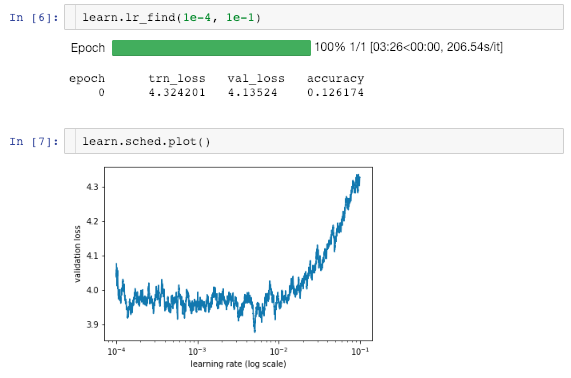# Unstable lr_find() Result

I try to deploy resnet34 model on my data and the basic configuration is similar to the one taught in lesson1. After a few training on last layer (precompute = False), I want to find the optimal learning rate again so I run lr_find() again. However, a strange result is shown as belows - the loss function fluctuate rapidly and look unstable in plot.

How should I decide the optimal learning rate from the plot?I find this is often the case; after some training there isn’t a clear minimum in the `lr_find` plot. You’d definitely want something less than 1e-2.

It seems in most of the example notebooks it’s only run at the start of the training and there’s some rule of thumb around how to decrease the learning rate in subsequent cycles (usually either kept constant or decreased by a factor of 2, 5 or 10).

Maybe try a few values (reloading each time, so you can check which is best at this point) and see what gives the best result. Post it back here; it would be great to know what the best choice is.